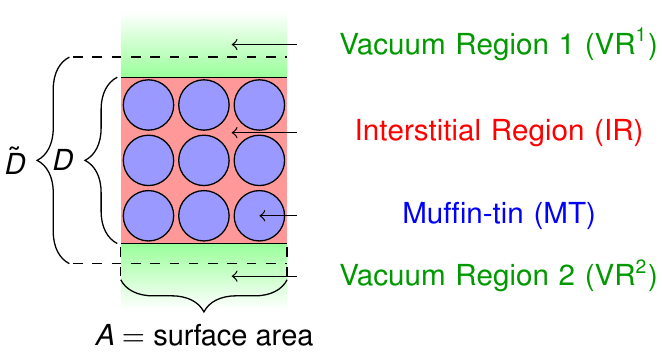## The LAPW basis for thin film systems

Calculations on thin films can be performed in different ways. A popular approach to this task is to define a bulk unit cell in which the film is provided along the xy-plane and the width of the film in z direction only covers a small fraction of the unit cell's length in that direction. Such a setup defines periodic repetitions of the film in z direction and one has to make sure that the vacuum in between the films is large enough to decouple them.

On the basis of the FLAPW method a more elegant treatment of thin film systems is possible. As sketched in figure 1 one can set up unit cells with semi-infinite vacuum regions above and below the film.An LAPW basis function for such a setup is defined as

where the extension in the vacuum regions consists of the two functions $u_{\vec{k}_\parallel \vec{G}_\parallel}^\text{vac}(z,E^\text{vac})$ and $\dot{u}_{\vec{k}_\parallel \vec{G}_\parallel}^\text{vac}(z,E^\text{vac})$ are solutions and energy derivatives to the Schrödinger equation in the respective vacuum region

at the energy parameter $E^\text{vac}$. The coefficients $a_{\vec{k}_\parallel\vec{G}}^{\text{vac}}$ and $b_{\vec{k}_\parallel\vec{G}}^{\text{vac}}$ are determined by enforcing continuity of value and slope of the basis function at the vacuum boundary defined by the parameter $D$. The z components of the plane waves in the interstitial region are constructed such that they feature a periodicty in agreement to the parameter $\tilde{D}$. Note that $\tilde{D}$ is larger than $D$ to avoid a kink of the implicitely periodic interstitial representation of the wave functions at the interstitial-vacuum boundaries.

For film setups the parameters $D$ and $\tilde{D}$ are specified in cell/filmLattice/@dVac and cell/filmLattice/@dTilda. For each vacuum the energy parameters are set in the respective cell/filmLattice/vacuumEnergyParameters element for the spin-up (cell/filmLattice/vacuumEnergyParameters/@spinUp) and spin-down (cell/filmLattice/vacuumEnergyParameters/@spinDown) channel separately, relative to the vacuum potential at an infinite distance from the film. In the case of a nonmagnetic calculation the value for the spin-up electrons is used for the calculation of the energy parameter. If both vacua are equivalent only a single cell/filmLattice/vacuumEnergyParameters element for vacuum 1 is present. It is then also used for vacuum 2.